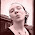## Friday, July 24, 2009

### A Class of Few

A class of students took a test. The results were mixed. One-third of the class received a B, one-fourth received a c, one-sixth received a D and one-eighth of the class flunked. Everyone else got an A.

How many students in the class got an A on the test (I'm not looking for a proportion here, but a real number).

Look below for a hint:

Hint: There is more than one possible answer, but if you restrict the class size to fewer than 30, you can find the first one.

Hint 2: Yes, this is a math question ;-)

#### 6 comments:

1.The number of kids in the class is 24n (24 is the lowest common multiple of the denominators of the fractions). 3n kids scored an A (what's left when you subtract all the other scores).

There are an infinite number of correct answers, unless you set an upper limit for class size. If that limit is 30, n=1 and 3 kids scored A's.

That was fun!

2.3.whatever number it is it has to be divided by 3,4,6,8 and not give a remainder. the smallest number that fits this condition is 24. so number of As = 24-8-6-4-3 = 3

4.funness :) i havnt done math all summer! i got 3 cuz all those numbers go into 24 (24 can be divided by all those numbers) so i made all the fractions hav the same denominator (24) and then added all those nominators and subtracted that number i got from 24 and got three :] if anyone understood that...im horrible at explanations.

5...~.~..MATH!..~.~..

..NEVER EVER FAILS to make the life of students DIFFICULT!!!..~.~..

..my answer is the same as the others though..^^

6.three people got an A. I figured that one out without trying to haha, I was only planning on writing the fraction of students that got an A and in the process of finding the same denominator I realized that the fraction 3/24 was the answer!!!!! Wow not as hard as I thought it was going to be lol :D

Leave your answer or, if you want to post a question of your own, send me an e-mail. Look in the about section to find my e-mail address. If it's new, I'll post it soon.

Please don't leave spam or 'Awesome blog, come visit mine' messages. I'll delete them soon after.

Enter your Email and join hundreds of others who get their Question of the Day sent right to their mailbox

Preview | Powered by FeedBlitz

The Lamplight Manor Puzz 3-DAre you looking for a particular puzzle, riddle, question, etc? Or do you want to find the answer today rather than wait till tomorrow!Web statspuzzles.blogspot.com